If a shape is divided into four equal parts it has been quartered.

# Year 2 Fractions - Shapes

This quiz addresses the requirements of the National Curriculum KS1 Maths and Numeracy for children aged 6 and 7 in year 2. Specifically this quiz is aimed at the section dealing with finding fractions of shapes.

Finding fractions of shapes means being able to understand that a shape split into two equal parts has been halved, that a shape split into four equal parts (perhaps by halving and then halving again) has been quartered and a shape split into three equal parts is in thirds. Children in Year 2 should also recognise the equivalence of two quarters equalling one half, and also that three quarters is greater than a half, but less than the whole. Using division is key when working out and finding fractions of whole numbers.

Question 1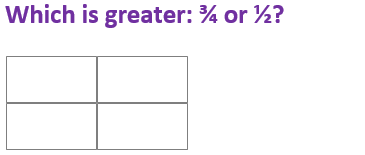24
They are both the same
12
34
12 is the same as 24 so 34 is greater
Question 2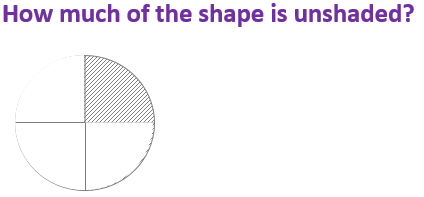24
34
44
14
3 of the 4 quarters are not shaded, only one quarter is shaded
Question 3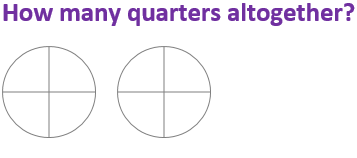8
6
5
10
Each shape has 4 quarters so there are 8 altogether
Question 4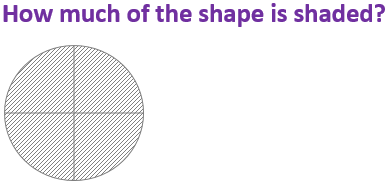24
14
44
12
The whole shape is shaded – this is 4 quarters
Question 5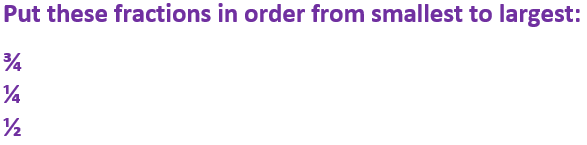34, 14, 12
14, 34, 12
12, 14, 34
14, 12, 34
12 is the same as 24
Question 6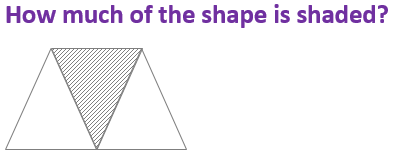23
14
13
12
One of the three equal parts is called a third
Question 7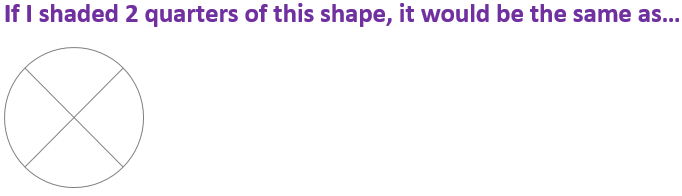12
34
25
14
There are four quarters. If I shaded two of them, it would be half of the shape
Question 8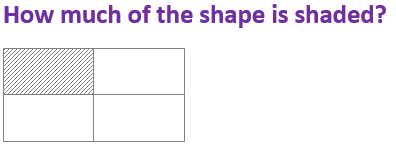24
14
12
34
The shape is divided into four equal sections – each one is a quarter. One is shaded
Question 9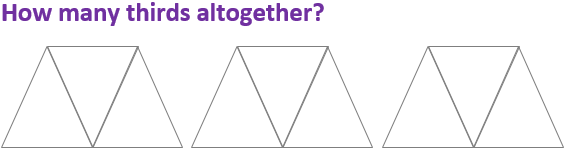5
3
6
9
Each shape has 3 thirds. There are 3 shapes so 3 x 3 = 9
Question 10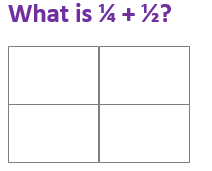24
14
34
12
If you shaded in 1 quarter, and then 2 more, you would have shaded 3 of the 4 quarters